## research papersJOURNAL OFSYNCHROTRONRADIATION
ISSN: 1600-5775
Volume 27| Part 2| March 2020| Pages 378-385

## Synchrotron radiation diffraction in a single crystal of paratellurite investigated with a new experimental scheme

aNational Research Centre Kurchatov Institute', 123182 Moscow, Russia, and bShubnikov Institute of Crystallography of Federal Scientific Research Centre Crystallography and Photonics' of Russian Academy of Sciences, 119333 Moscow, Russia
*Correspondence e-mail: kohnvict@yandex.ru, ontonic@gmail.com

(Received 11 September 2019; accepted 25 December 2019; online 7 February 2020)

First results are presented for synchrotron radiation diffraction in a paratellurite (TeO2) single crystal investigated with a new experimental scheme consisting of a standard monochromator and a relatively narrow slit for collimation and monochromatization of an incident beam. The Bragg case reflection geometry is used. The monochromator, a pair of Si crystals, maintains the initial direction of the beam propagation. The theory is developed for a precise description of the instrumental function in such a scheme. A new rocking curve registration technique with use of an adaptive bending piezoactuator is applied for the first time with the aim to record the narrow diffraction peaks with high accuracy. A sample is attached to one edge of the bidomain lithium niobate single crystal piezoactuator used. The piezoactuator is bent under the influence of an electric field and it changes the angular position of the sample with a very small step. The experimental curves are compared with the calculated diffraction rocking curves of a perfect paratellurite crystal, both the theoretical one and the one calculated taking into account the instrumental function. An instrumental function account allows a complete coincidence of the simulated curves with the experimental results to be obtained if the experimental curve does not coincide with the theoretical rocking curve. A small discrepancy between the theory and the experimental data has been discovered in cases where the incident beam is sufficiently collimated and monochromated. This indicates the presence of a certain number of lattice defects in the sample under study.

### 1. Introduction

X-ray diffraction on a three-dimensional periodic lattice of atoms in a single crystal is one of the most beautiful effects of solid-state physics. The simplest and purest case takes place for a plane monochromatic incident wave. For this case, the theory was first presented by Darwin in 1912, then by Ewald in 1917, and later Laue developed the modern theory (Authier, 2005).

However, the diffraction theory has long been difficult to use, since most natural crystals are polycrystals. They consist of very small crystallites, between which the lattice is distorted. The only exceptions are some minerals of natural quartz. In the 1960s, large single crystals were created artificially as basic elements of modern microelectronics.

At the same time, methods of X-ray beam collimation and monochromatization were developed. For laboratory X-ray sources, the fact that the atomic characteristic radiation is initially quasi-monochromatic with an effective full width at half-maximum (FWHM) of the spectrum of ΔE/E ≃ 300 µrad was useful. For two-wave diffraction in the Bragg case (reflection geometry), it is sufficient to use two crystals in a non-dispersive scheme and record the intensity dependence on the rotation angle of the second crystal (sample) relative to the first crystal (monochromator).

With use of asymmetric reflection in a monochromator, it is possible to obtain an almost theoretical diffraction rocking curve (DRC). For crystals with defects, a three-crystal scheme is used to analyze an angular spectrum of the reflected radiation and to isolate the diffuse scattering. However, there are still problems in the study of multiple diffraction even in the coplanar scheme, since the second reflection is highly distorted by the instrumental function (Blagov et al., 2010).

In the case of synchrotron radiation (SR), the situation is complicated by the fact that the incident radiation has a very wide spectrum and one monochromator crystal is insufficient. For high-precision measurements of multiple diffraction DRCs, a multi-crystal monochromator collimator is required (Kazimirov & Kohn, 2010, 2011; Kohn & Kazimirov, 2012).

At the experimental station RKFM of the Kurchatov Source of Synchrotron Radiation in Moscow, Russia, a double-crystal monochromator is installed together with a two-dimensional slit. The monochromator does not change the direction of the SR beam propagation. The first work (Blagov et al., 2011) on the study of coplanar three-beam diffraction showed that the DRC of the second reflection is broadened even in a non-dispersive scheme.

Nevertheless, such a relatively simple scheme sometimes allows one to record almost theoretical DRCs with the use of SR even in the dispersion scheme. This was first shown theoretically (Kohn, 2018; Kohn, 2019a) and experimentally (Kohn et al., 2019). At that time, however, another problem remained, namely that the angle step in the standard experimental scheme is equal to 0.0002° = 3.49 µrad, which does not allow theoretical DRCs to be recorded, especially for high orders of reflection, with sufficient accuracy.

This problem was solved by using a new method of DRC detection based on an adaptive piezoactuator (Bykov et al., 2014; Blagov et al., 2016, 2017; Eliovich et al., 2018; Kulikov et al., 2019; Marchenkov et al., 2019), which allows the DRCs to be recorded in increments of 0.01 µrad or less. In this work, a new experimental scheme is used to study SR diffraction in a single crystal of paratellurite (TeO2). The scheme is based on a two-crystal monochromator and a slit, as well as a new method of DRC detection.

A combination of three reflections in the monochromator and four reflections in the sample were investigated with the aim to show the parameters at which the new scheme gives adequate results, namely gives theoretical DRCs. For this purpose, all experimental curves are compared with the theoretical curves computed according to the theory developed by Kohn (2018, 2019a). The next section describes the experiment. In the following sections the theory and results of comparisons of the experimental DRCs with the theory are presented.

### 2. Experiment

#### 2.1. Sample and method of angular measurement

The sample was a single crystal of paratellurite (α-TeO2) with dimensions of 15 mm × 10 mm and a thickness of 1.15 mm. The surface was parallel to the (110) atomic planes. High-quality single crystals of paratellurite were grown at the Federal Scientific Research Centre (FSRC) Crystallography and Photonics' of the Russian Academy of Sciences . The deviation of the sample-surface normal direction and the crystallographic direction  did not exceed 0.03°. For the experimental study, the surface of the sample was prepared using a fine asymptotic grinding technique and etched in a 25% solution of hydrofluoric acid in order to remove the disrupted layer.

For precision angular scanning of the DRCs, a new technique based on an adaptive piezoactuator was used. This piezoactuator was a bidomain single crystal of lithium niobate (LiNbO3), 40 mm in length and 1 mm in thickness with Y+128° cut (rotation of the Cartesian coordinate system along the x axis). To one edge of the bidomain piezoactuator the sample under study was bounded. Fig. 1shows a photograph of the sample with the piezoactuator fastened to the holder.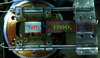Figure 1 Photograph of the adaptive element (piezoactuator) with the bounded sample. The length of the scale bar under the TeO2 sample is 1.5 cm.

The piezoactuator was manufactured at NUST MISiS', Moscow, Russia. The production technology of such elements was described in detail by Bykov et al. (2014). The lithium niobate crystal was subjected to a specialized laser annealing, leading to the formation of a domain wall at half of the thickness dividing the crystal into two parts with opposite polarization direction.

Application of the control electric signal to the front surfaces activates the piezoelectric module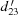(in the rotated Cartesian coordinate system), which leads to the bending of the whole crystal. The value of the bending depends on the external field strength. Thus, when an electric signal is applied to the piezoactuator, it performs a controlled θ scan by varying the beam incidence angle on the sample.

The piezoactuator was console-type fixed in the crystal holder with the possibility of applying control direct-current or alternating-current electrical signals (Fig. 2). Descriptions of the fastening design, functional characteristics and features of the piezoactuator usage in X-ray diffraction experiments are given elsewhere (Blagov et al., 2016, 2017; Kulikov et al., 2019; Marchenkov et al., 2019). It was also used as a fast-tunable adaptive element for in situ study of the external mechanical stress in crystals by X-ray diffraction in the experiment with time resolution (Eliovich et al., 2018).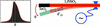Figure 2 Scheme of the measuring system consisting of a bidomain lithium niobate piezoactuator and a paratellurite single crystal under study.

The crystal holder with fastened piezoactuator and sample were installed on a 4+2'-circle goniometer system (HUBER). This system allows precision adjustments to be made.

#### 2.2. Experimental scheme

The experiment was performed at the Kurchatov Source of Synchrotron Radiation on experimental station RKFM. The basic configuration of the optical scheme of the station (Fig. 3) was used, which has been described in detail by Kohn et al. (2019). The parameters of the experiment were as follows: l0 = 13 m, effective source size wx = 2.355σx and σx = 54 µm. The distances l1 and l2 do not influence the results.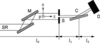Figure 3 Sketch of the experimental scheme. SR = synchrotron radiation, M = monochromator, S = slit, C = sample crystal and D = detector.

The station is equipped with a double-crystal FMB Oxford monochromator (M) with the ability to fine-tune the angular position of the second crystal (pitch). Two pairs of silicon crystals with 111 and 311 cuts are available for use.

The radiation source is a bending magnet. The spectral intensity distribution of the source has a maximum at 10–12 keV. The radiation energy used was E = 13.6 keV (λ = 0.91165 Å). The monochromator was tuned to the reflections 111, 311 and 333.

For the selected radiation energy, the Bragg angle, θB1, of reflection in the monochromator as well as the FWHM of the theoretical DRCs, ΔθM, can be calculated via online computer program (Kohn, 2019b). Accordingly, we obtain the values θB1 = 8.359, 16.163 and 25.857° and ΔθM = 19.3, 7.8 and 4.0 µrad for reflections 111, 311 and 333, respectively. After the monochromator, the beam was collimated by a two-dimensional slit (S), the size of which was set with an accuracy of 0.005 mm. The aperture of the vertical slits (along the x axis in accordance with Fig. 3) was 0.1 mm and the aperture size of the horizontal slits (along the y axis) was 1.0 mm.

The experimental DRCs were recorded for four symmetric reflections, 110, 220, 330 and 440, of the sample, whose Bragg angles are equal to 7.702, 15.548, 23.707 and 32.417°, respectively. The sample was sequentially adjusted in diffraction conditions for the given reflections. The standard way to record a DRC is by θ scan, by rotating the goniometer with the sample near the Bragg position in the reflection geometry using a step motor. In this technique the minimal scanning step was 0.0002° (3.49 µrad). The radiation intensity was recorded using a Bruker scintillation NaI detector, in front of which a pneumatic beam attenuation system with a set of aluminium and copper foils was installed.

It should be noted that the DRC FWHM of perfect single crystals, especially for high reflection orders, which is often the case in the study of multiple diffraction (Blagov et al., 2010, 2011; Kazimirov & Kohn, 2010, 2011; Kohn & Kazimirov, 2012), can be equal to several microradians or less. For high-quality registration of such a DRC scan step, 0.0002° is insufficient and this registration scheme cannot be used.

In view of this, a new technique for accurate DRC registration using a bending piezoactuator in the quasi-static operational mode, described in detail by Kulikov et al. (2019), was used in this work. In this mode, the piezoactuator receives a low-frequency triangle control electrical signal (0.5 Hz in this experiment). At the same time, intensity was registered depending on the phase of the oscillations.

It is worth noting that modern generators allow the generated signal parameters profile to be drawn with high precision. This gives the opportunity to control the X-ray beam promptly in some range without a lack of discrete angular tuning which is inherent for step motors.

The intensity is accumulated by counting transistor–transistor logic (TTL) pulses from the scintillation detector using an ORTEC Easy MCS (multichannel scaler), which is synchronized with the control signal from a Rigol DG4062 generator. The MCS distributes the intensity through the short time channels. The bending angle of the piezoactuator (along the θ axis) depends linearly on the channel number or, in other words, the phase of the control signal, which makes processing of the collected data as convenient as possible.

The maximum speed of this registration technique is achieved when the whole DRC is recorded for half of the oscillation period. The minimal scanning step Δθ is determined by the ratio of the amplitude of the angular shift of the piezoactuator W to the number of channels N of the MCS, i.e. Δθ = 2W/N. If better statistics are necessary, registration of the DRC is possible for several oscillation periods. In this case, there are multiple photons' acquisition periods in each channel while the channel number corresponds to a certain phase of oscillation.

The proposed technique allows the existing goniometric systems for precision experiments to be modernized without the need to increase the angular accuracy with the help of complex mechanics, since only one module with an adaptive bending element (piezoactuator) and an electric signal generator are necessary. It is worth noting that the piezoactuator used in this work was hysteresis free, in contrast to the widely used piezoceramic actuators and stages. Thus, the application of the proposed technique will significantly expand the functionality of the existing experimental equipment, for both laboratory and synchrotron sources.

### 3. Theory

The theory of two-wave diffraction of a plane monochromatic X-ray wave in a perfect crystal is described in detail in textbooks [see, for example, Authier (2005)]. However, in experiments it is almost impossible to create a plane monochromatic wave. In reality, various schemes are used to restrict the spectrum of radiation incident on the crystal in the energy scale as well as in the beam-divergence scale.

In this work, for the experiments using the SR source, a scheme with a two-crystal monochromator and a slit in the vertical plane was used (see Fig. 3). This scheme is suitable for studying both two-wave diffraction and multiple diffraction in the coplanar case. The theory of coplanar multiple diffraction taking into account the instrumental function for this scheme of the experiment was developed by Kohn (2018).

It was shown that in such a scheme and in the case of coplanar multiple diffraction of X-rays the reflected beam intensity with an arbitrary normalization is described by the matrix S(θr, θ1), where θr is the crystal rotation angle with respect to the beam and θ1 is the rotation angle of the monochromator, which sets the desired average energy of photons. This matrix is determined by the convolution of the theoretical matrix of the angular and energy dependence of the plane wave reflection GC(θr, θ1) and the matrix of the instrumental function H(θ, θω), which determines the angular and energy spectrum of the incident radiation. This may be written as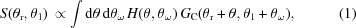where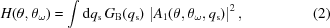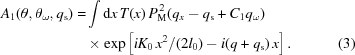Here q = K0θ, qω = K0θω, qs = K0xs/l0, qx = K0x/l0, C1 = tan(θB1), θB1 is the Bragg angle for the reflection of the monochromator, PM is the monochromator reflection amplitude,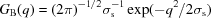and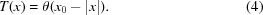The parameter σs = K0σx/l0 defines the effective transverse size of the source, x0 is half of the slit width, l0 is the distance from the source to the slit, K0 = (2π/hc)E0 and E0 is the average photon energy in the incident beam, which is obtained at a given monochromator rotation angle θ1.

Equation (1) is a convolution of two functions in the plane of two variables: the angular deviation θ and the relative energy deviation θω. This plane is widely used for creating a DuMond diagram in the case of a multi-crystal monochromator for experiments with a laboratory source. It is assumed in this approach that the incident radiation can be approximated by a superposition of incoherent plane waves with infinite angular and energy spectrum, and several crystals can create a finite area of non-zero intensity as the instrumental function H(θ, θω).

This area arises as the area of intersection of stripes of finite width which represents the total reflection area for each crystal. In the case of a single-crystal monochromator the DRC will have infinite width in all cases except for the non-dispersive case when the Bragg angle in the monochromator and in the sample are equal to each other.

In our case the instrumental function H(θ, θω) is calculated in quite a different way. The scattering of radiation by a double-crystal monochromator is taken into account coherently. The diffraction of radiation on a slit is seen as a coherent process. Equations (2) and (3) cannot be represented by a DuMond diagram in general cases.

In this work we restrict ourselves to the simpler case of a very thin slit when the function H(θ, θω) has the form of the product of two functions (see below). One of the functions is the total reflection area for the monochromator crystal; the other function does not depend on θω and has a finite width along the θ axis. Such a case can be represented by an analog of DuMond diagram. However, we will show that there is a simpler way to calculate the results.

In the two-wave case, the reflection in the sample depends on only one parameter, θr + C2θ1, where C2 = tan (θB2) and θB2 is the Bragg angle for reflection in the sample. Let us consider the case when the slit is small enough that the curvature of the wavefront in the slit region can be neglected. On the other hand, the size of the source exceeds the size of the slit, so that one can neglect the size of the slit in the reflection function of the monochromator.

In the specified limit case, equation (3) can be approximately rewritten as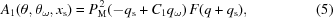where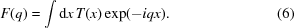Let us introduce new functions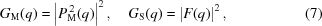and consider their Fourier transforms in direct space, namely, present them as integrals,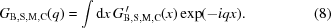Substituting equations (5)–(8) into equations (1) and (2) we obtain a seven-fold integral. However, the three integrals are equal to Delta functions, which remove three more integrals, and the final equation can be written in the form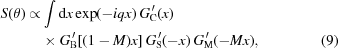where M = C2/C1. Note that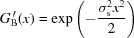and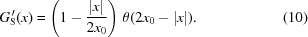Equation (9)makes the calculation very easy, given that there is a fast Fourier transform procedure. As will be shown below, it yields results that are in good agreement with experiment. This equation was first proposed by Kohn et al. (2019).

### 4. Results of the first series of experiments

The first series of experiments was performed with the Si 111 reflection of the monochromator. This is a standard monochromator that can be found at almost all stations of SR sources. It gives the maximum energy interval at the natural angular divergence of the beam. For many experiments a very high monochromatization is not necessary and therefore a weak monochromatization is used without loss of integral radiation intensity.

In general, however, monochromatization is necessary because SR has a very wide bandwidth. For diffraction experiments, such a monochromator gives a very poor resolution, insufficient to register the angular dependence of the high-order reflections of the sample.

A photon energy of E = 13.6 keV (θB1 = 8.359°) was used. Fig. 4shows the DRCs for reflections 110 with Bragg angle θB2 = 7.702°, 220 with θB2 = 15.548°, 330 with θB2 = 23.707° and 440 with θB2 = 32.417°. For the reflection TeO2 110, the parameter M = tan(θB2)/tan(θB1) = 0.92 and the DRC is wider, since the polarizability of Te is large compared with Si. For this reason, the measured curve is close to the theoretical curve.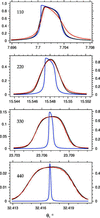Figure 4 Comparison of the theoretical DRC (blue), the DRC with instrumental function (black) and the experimental DRC (red) in the first series of measurements. The X-ray beam reflection in the monochromator is 111; the reflection in the sample is indicated in each panel. Values of the parameter M are 0.92, 1.89, 2.99 and 4.32 for the panels from top to bottom, respectively.

In this figure, as well as in Figs. 5 and 6, the theoretical DRC is shown in blue, the theoretical DRC taking into account the instrumental function is shown in black and the experimental DRC is shown in red. The curves are drawn in a specific order such that the experimental one is visible in case of overlap. It should be noted that the experimental curves were not normalized and the Bragg angles for the experimental curves were slightly different compared with calculated values obtained from the known lattice parameters and the radiation wavelength.

In the calculation we used the values of the diffraction parameters, i.e. the Fourier component of the polarizability of the monochromator and the sample, as well as the geometric parameters which are given by online computer program (Kohn, 2019b). We found the position of each experimental curve center as well as the maximum value from the condition of the best visual coincidence of the calculated and experimental curves.

It should also be noted that the experimental DRC contained a lot of points (>5000), but they were not smooth due to noise effects. To eliminate the noise the data were first summarized every nine points and then smoothed by replacing each point by the sum of its neighbors (50% to the current point itself and 50% to the neighbors). The latter procedure was repeated several times until smooth curves as shown in Figs. 5, 6 and 7 were obtained. The raw and smoothed DRCs are discussed in Appendix A.

As can be seen in Fig. 4, in this case the calculated curve and the experimental curve do not completely coincide. There are small differences, especially on the right-hand slope of the peak. The reason for this is that the experimental curve is close to the theoretical DRC of the sample and just gives information about the sample imperfections, such as the weak deformations of the crystal lattice, the nature of which is difficult to determine. However, all calculations were performed for a perfect single crystal.

For the TeO2 220 reflection, the parameter M = 1.89 is greater than 1. For this reason the instrumental function significantly distorts the theoretical DRC. For a better representation of this and subsequent figures the theoretical curve corresponds to the right-hand ordinate axis whereas the experimental curve and the theoretical curve taking into account the instrumental function correspond to the left-hand ordinate axis, the values of which can be significantly less.

It is easy to see that taking into account the instrumental function in the calculated curve leads to very good matches with experiment. But both curves are noticeably broadened and their shape corresponds to the angular divergence of the incident beam taking into account its non-monochromaticity, which is determined by the monochromator unit, the source and the slit, but not by the sample.

For the TeO2 330 reflection, the experimental curve again matches well with the theoretical one that includes the instrumental function contribution. Hence, the diffraction results contain even less information about the sample. It is interesting that the areas under the theoretical DRC and under the DRC with instrumental function are approximately equal because the instrumental function is normalized to a unit area.

For the TeO2 440 reflection, the instrumental function significantly spoils the curve. The DRC that includes the instrumental function contribution has a very large width and about the same area. Accordingly, it has a very low height. The correspondence between theory and experiment is again very good. However, in this case the experimental curve showed artifacts that could not be completely eliminated even by the noise-elimination procedure. On the other hand, the experimental curve is symmetric, but the theoretical curve is not. It is possible that the theory in this case is not quite accurate, since the approximation of a thin slit is used.

As a result, it is possible to make the general statement that when the instrumental function distorts its theoretical curve the experimental curve matches better with the theory taking into account the instrumental function even in the not very accurate case of a thin-slit approach. It is easy to understand from the considerations that the distortion is mainly produced by the silicon monochromator and its perfection guarantees the accuracy of the calculation of its theoretical DRC. Thus, these results clearly show that the experimental scheme with monochromator and slit gives reasonable data close to the theoretical curves only when the Bragg angle for the monochromator reflection is greater than the Bragg angle for the sample reflection, that is, for M < 1. This conclusion of the theory is definitely confirmed by the experimental results.

### 5. Results of the second series of experiments

In the second series of experiments, the Si 311 reflection of the monochromator was used with the Bragg angle θB1 = 16.1635°. The results are presented in Fig. 5. In this case, for the TeO2 110 reflection, the theoretical curve taking into account the instrumental function almost coincides with the theoretical one (M = 0.47). The experimental curve is very close to that shown in Fig. 4, but it differs more from the theoretical curve even taking into account the instrumental function.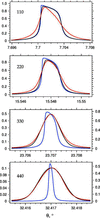Figure 5 Comparison of the theoretical DRC (blue), the DRC with instrumental function (black) and the experimental DRC (red) in the second series of measurements. The X-ray beam reflection in the monochromator is 311; the reflection in the sample is indicated in each panel. Values of the parameter M are 0.47, 0.96, 1.52 and 2.19 for the panels from top to bottom, respectively.

For the TeO2 220 reflection the situation is close to the 110 reflection in the previous case (M = 0.96). However, the experimental curve differs slightly from the theoretical curve in other ways than in the above case. The reason for this could be the difference in the diffraction parameters of the sample.

For the TeO2 330 reflection the theoretical curve with the instrumental function differs significantly from the theoretical DRC (M = 1.52) and, as a consequence, it matches better with the experimental curve. This means a loss of information about the structure distortions in the sample.

For the TeO2 440 reflection the instrumental function spoils the DRC even more (M = 2.19), and takes the standard form of the convolution of the source, monochromator and slits functions, so the theoretical DRC of the sample is almost not noticeable.

### 6. Results of the third series of experiments

In the third series of experiments, the Si 333 reflection of the monochromator was used with the Bragg angle θB1 = 25.858°. The results are presented in Fig. 6. In this case, for the TeO2 110 reflection, the theoretical curve with the instrumental function matches even better with the theoretical curve (M = 0.28). However, the experimental curve has not changed much and is similar to the one shown in the previous figure. It is safe to say that the reflection in the sample does not correspond to the theory for a perfect crystal, although the difference is not very large.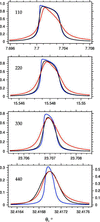Figure 6 Comparison of the theoretical DRC (blue), the DRC with instrumental function (black) and the experimental DRC (red) in the third series of measurements. The X-ray beam reflection in the monochromator is 333; the reflection in the sample is indicated in each panel. Values of the parameter M are 0.28, 0.57, 0.91 and 1.31 for the panels from top to bottom, respectively.

For the TeO2 220 reflection, the situation is intermediate between the theoretical curve with the instrumental function and the theoretical curve of the 110 reflection (M = 0.57). The reason for this could be the difference in the diffraction parameters of the sample as well as a small influence of the radiation spectrum caused by incomplete collimation and monochromatization.

For the TeO2 330 reflection, the theoretical curve with the instrumental function is still very close to the theoretical one (M = 0.91) and, as a consequence, it does not match with the experimental curve. It is interesting that the difference between the curves is more significant than for weaker reflections. This is easy to understand because a displacement of atoms from their positions in a perfect crystal u caused by crystal lattice deformation influences the DRC through the phase shift φ = hu, where h is the reciprocal lattice vector of reflection. This phase shift is taken into account in the Takagi equations (Authier, 2005). Therefore, the larger the modulus of h, the larger the phase shift. Thus, on the basis of these data it can be concluded that there are weak deformations in the paratellurite sample.

For the TeO2 440 reflection, the instrumental function spoils the DRC even more (M = 1.31) and the experimental curve is already close to the theoretical one with the instrumental function. Here, information about the deformations in the crystal is lost.

### 7. Conclusions

This article presents the results of an experimental study of SR diffraction in a paratellurite single crystal using a new experimental scheme. Collimation and monochromatization of the beam incident on the sample is performed using a double-crystal monochromator and a two-dimensional slit. Narrow rocking curves were measured using an adaptive piezoactuator non-mechanically. Experimental DRCs are compared with two theoretical DRCs: the proper one and one that takes into account the instrumental function, such as the influence of the source, monochromator and slit sizes.

In an extensive series of measurements with different monochromator and sample reflections the full accordance of the experiment and theory taking into account the instrumental function is convincingly shown in cases when the instrumental function significantly distorts the theoretical DRC. This occurs when the Bragg angle in the sample is greater than the Bragg angle in the monochromator and the parameter M > 1.

In the opposite case (M < 1), almost theoretical DRC of the sample containing the most useful information about the sample is obtained in the experiment. In this case, the theoretical DRC did not completely match the experimental one, that can be connected with the presence of the weak crystal lattice deformations and other imperfections in the sample under study. This fact is also indicated by the deviation increasing with the reflection order.

Thus, the proposed experimental scheme can be used to obtain the theoretical DRC of the sample in both the two-wave case and the coplanar multiple case of diffraction only by using high reflection orders in a monochromator.

### Raw experimental data

The raw experimental DRC for the first series of measurements contained 5001 points in the interval from 7.6947 to 7.7113°. Fig. 7shows the smaller interval from 7.6959 to 7.7081° which is also shown in Fig. 4. The raw DRC contains 3676 points and is shown in black. The smoothed DRC contains 408 points for the same interval and is shown in green. A procedure for noise elimination allows a clearer difference to be seen between the experimental and theoretical DRCs.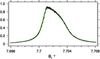Figure 7 Comparison of the raw DRC (black) and the smoothed DRC (green) of Fig. 4, top panel. The case of the 110 reflection in the sample and the 111 reflection in the monochromator is presented.

### Funding information

This work was supported by the Ministry of Science and Higher Education within the State assignment FSRC Crystallography and Photonics' of RAS in part of crystal growth and sample preparation' and by the Russian Foundation for Basic Research (project 19-29-12043mk and 18-32-20108mol_a_ved) in part of collecting the experimental data'.

### ReferencesJOURNAL OFSYNCHROTRONRADIATION